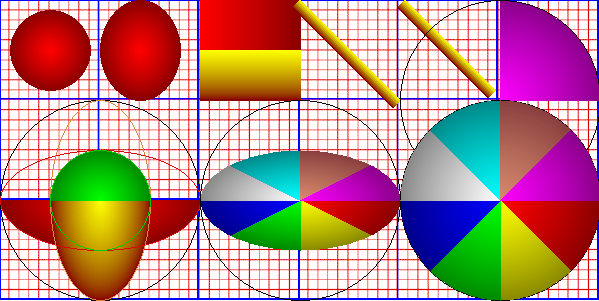The radial gradients revolve around a center point. The first red radial is placed at 50,50 and is 80,80 in width and height, so it appears centered in the 100x100 grid space, with 10 pixels of space all around the gradient, making it 80x80 in size. The second radial is placed at 140,50, and is 80,100 in wxh. It is 40 pixels in from the left and is 80 pixels wide which makes it flush against the left side of the block. Being 100 in height and placed at 50 on the y axis, makes it fill the square. The red horizontal gradient placed at 200,0 is 100x50 in size and is placed where expected. The yellow vertical gradient is placed at 200,50 and is 100,50 in size and is placed where expected. The first angle is placed at 300,0 to 400,100 and is 10 in width. It appears a little off center as it begins to draw at the points specified. To move it more to the corners, the second angle is placed at 405,0, to 495,90. The topmost arc on the right is placed at 500,100 and is 200, 200 in size with an arc of 270,0. The arcs revolve around a center point (500,100) and is assuming the radius of the circle is 200,200, so the arc only draws out at a size of 100x100. the circle drawn around the arc is 200x200.More arc studies. Bottom left box of arcs show a red arc placed at 100,200, size of 200,100, arc of 0,180. The green and yellow arcs are also placed at the center point of 100,200, but the yellow arc is 100x200 in size arc of 0,180. The green arc is 100x100 in size and arc of 180,0. The middle bottom arcs are all placed at 300,200 (assuming a circle of 200,100 which is the size of all arcs). The circle drawn around them is 200x200. The arcs on the bottom right are all drawn at 500,200, all are 200,200 in size. The arcs beginning at the red arc run from 0,45, yellow 45,90, green 90,135, blue 135,180, white 180,225, aqua 225,270, tan 270,315, magenta 315,0. Blank grid